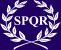# Census MMDCCLXIV Registered

(Difference between revisions)
Jump to: navigation, search
 Revision as of 18:21, 23 June 2011 (view source)m (Protected "Census MMDCCLXIV Registered": Census page [edit=sysop:move=sysop])← Older edit Revision as of 18:23, 23 June 2011 (view source) (censors banner)Newer edit → Line 1: Line 1: + {{Censores}} + + __TOC__ __TOC__

## Revision as of 18:23, 23 June 2011This page is maintained under authority of the Censores. Make no unauthorized changes .

## Nova Roman provinces

 Nova Roman provinces Registered citizens Paulla Corva Gaudialis Total= Helena Galeria Aureliana Gaius Popillius Laenas Gaius Fulvius Severus Total= Total= Lucia Cornelia Ursula Total= Total= Total= Titus Marcius Felix Lucius Arminius Lupercus Total= Gaius Marcius Crispus Total= Aulus Liburnius Hadrianus Total= Registered citizens Gnaea Livia Ocella Aulus Sallustius Barbatus Total= Total= Decimus Marius Corvinus Gaius Tullius Niger Total= Diana Octavia Aventina Quintus Apollonius Iustus Total= Total= Total= Decimus Gladius Lupus Total= Publius Cornelius Optatus Total= Total= Registered citizens Total= Gaius Aemilius Priscus Marcus Cornelius Dexter Gaius Quinctius Flamininus Gaius Cassius Vespa Gallio Velius Marsallas Total= Total= Total= Total= Total= Total= Publius Candidianus Botius Total= Total=

## Countries with Nova Roman presence

 Countries; territories w/Nova Roman presence Registered citizens Bulgaria Total= Chile Total= China Total= Colombia Total= Ecuador Total= Estonia Total= Greece Total= Croatia Total= Israel Total= Registered citizens India Total= Jamaica Total= Jordan Total= Lebanon Total= Lithuania Total= Macedonia Total= Nigeria Total= Peru Total= Puerto Rico Total= Quatar Total= Registered citizens Saudi Arabia Total= Singapore Total= Slovenia Total= Thailand Total= Turkey Total= Taiwan Total= Venezuela Total= Serbia Total= South Africa Total=

Census page.

Statistics page